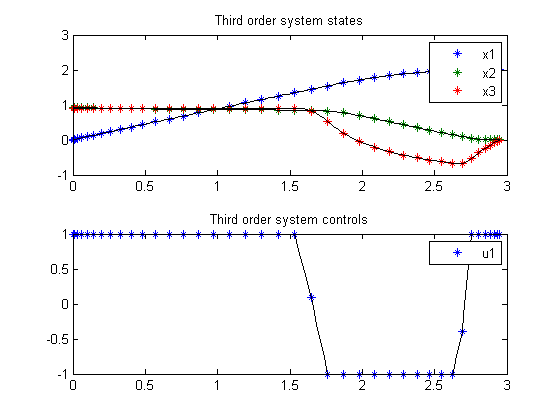112  Third order system

112.1  Problem description

Time-optimal control of a third order system with bounded control.

Programmers: Gerard Van Willigenburg (Wageningen University) Willem De Koning (retired from Delft University of Technology)

112.2  Problem setup

% Array with consecutive number of collocation points
narr = [20 40];

toms t t_f % Free final time

for n=narr
p = tomPhase('p', t, 0, t_f, n);
setPhase(p)

tomStates x1 x2 x3
tomControls u1

% Initial & terminal states
xi = [0; 0.931; 0.9];
xf = [2;     0;   0];

% Initial guess
if n==narr(1)
x0 = {t_f == 5; icollocate({x1 == xi(1); x2 == xi(2)
x3 == xi(3)})
collocate({u1 == 0})};
else
x0 = {t_f == tfopt; icollocate({x1 == xopt1; x2 == xopt2
x3 == xopt3})
collocate({u1 == uopt1})};
end

% Box constraints
cbox = {-1 <= collocate(u1) <= 1};

% Boundary constraints
cbnd = {initial({x1 == xi(1); x2 == xi(2); x3 == xi(3)})
final({x1 == xf(1); x2 == xf(2); x3 == xf(3)})};

% ODEs and path constraints
dx1 = x2;
dx2 = -x2-0.1*x2.*x2.*x2+x3;
dx3 = -2*x3+-0.2*x3./sqrt(x3.*x3+1e-4)+2*u1;

ceq = collocate({
dot(x1) == dx1
dot(x2) == dx2
dot(x3) == dx3});

% Objective
objective = t_f;

112.3  Solve the problem

options = struct;
options.name = 'Third order system';
solution = ezsolve(objective, {cbox, cbnd, ceq}, x0, options);

tfopt = subs(t_f,solution);
xopt1 = subs(x1,solution);
xopt2 = subs(x2,solution);
xopt3 = subs(x3,solution);
uopt1 = subs(u1,solution);
Problem type appears to be: lpcon
Starting numeric solver
===== * * * =================================================================== * * *
TOMLAB - Tomlab Optimization Inc. Development license  999001. Valid to 2011-02-05
=====================================================================================
Problem: ---  1: Third order system             f_k       2.956507317430983900
sum(|constr|)      0.000000000033334328
f(x_k) + sum(|constr|)      2.956507317464318200
f(x_0)      5.000000000000000000

Solver: snopt.  EXIT=0.  INFORM=1.
SNOPT 7.2-5 NLP code
Optimality conditions satisfied

FuncEv    1 ConstrEv   12 ConJacEv   12 Iter    9 MinorIter  101
CPU time: 0.046875 sec. Elapsed time: 0.047000 sec.
Problem type appears to be: lpcon
Starting numeric solver
===== * * * =================================================================== * * *
TOMLAB - Tomlab Optimization Inc. Development license  999001. Valid to 2011-02-05
=====================================================================================
Problem: ---  1: Third order system             f_k       2.949634637798719300
sum(|constr|)      0.000001841572751425
f(x_k) + sum(|constr|)      2.949636479371470900
f(x_0)      2.956507317430983900

Solver: snopt.  EXIT=0.  INFORM=1.
SNOPT 7.2-5 NLP code
Optimality conditions satisfied

FuncEv    1 ConstrEv    5 ConJacEv    5 Iter    4 MinorIter  136
CPU time: 0.062500 sec. Elapsed time: 0.063000 sec.
end

figure(1)
subplot(2,1,1);
ezplot([x1; x2; x3]); legend('x1','x2','x3');
title('Third order system states');

subplot(2,1,2);
ezplot(u1); legend('u1');
title('Third order system controls');